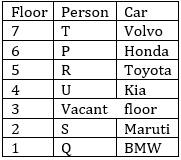Latest Banking jobs   »

# Reasoning Ability Quiz For SBI PO Prelims 2022- 14th December

Directions (1-5): Study the information carefully and answer the questions given below.
Six persons P, Q, R, S, T and U are living at seven storey building. Each of them like different Cars i.e., Maruti, Honda, Kia, BMW, Toyota and Volvo. All information is not necessarily in same order. One floor is vacant
P live at 6th floor. One floor is between P and the one who likes Kia. Two persons live between the person who likes Honda and the one who likes Maruti. The person who likes Honda lives above the one who likes Maruti. Q likes BMW and lives at bottom floor. R lives immediate below P’s floor and does not like Honda. Two persons live between U and T. One person lives between T and the one who likes Toyota. Vacant floor is one of the above floors of the person who likes Maruti.

Q1. Who among the following person likes Maruti car?
(a) P
(b) S
(c) Q
(d) T
(e) R

Q2. Which of the following floor is Vacant?
(a) 2nd
(b) 7th
(c) 5th
(d) 1st
(e) None of these

Q3. Which of the following combination is true?
(a) R-BMW
(b) P-Toyota
(c) U-Kia
(d) S-Honda
(e) Q-Maruti

Q4 Four of the following five are alike in certain way based from a group, find the one that does not belong to that group?
(a) Q-R
(b) U-Q
(c) T-R
(d) U-P
(e) S-R

Q5. Who among the following person likes Volvo car?
(a) P
(b) S
(c) Q
(d) T
(e) R

Directions (6-10): These questions are based on the following six numbers.

312 876 156 285 734 954

Q6. If we add 2 to the second digit of each number and subtract 1 from the first digit of each number, then which of the given will become the lowest number?
(a) 312
(b) 876
(c) 156
(d) 285
(e) None of these

Q7. If all the digits in each of the numbers are written in increasing order within than number, then which of the given number will become the second highest?
(a) 312
(b) 954
(c) 156
(d) 285
(e) None of these

Q8. If the first two digits of each number are interchanged, then which of the following numbers will become the highest number?
(a) 721
(b) 876
(c) 937
(d) 387
(e) 285

Q9. In each number, if we subtracted 1 in first digit and added 1 in last digit, then which among these number will be 2nd lowest number?
(a) 734
(b) 876
(c) 954
(d) 285
(e) None of these

Q10.If we replace all the odd digits in each number with zero, then which of the following number will become the lowest?
(a) 312
(b) 876
(c) 156
(d) 285
(e) 734

Direction (11-15): Study the following alphanumeric series carefully and answer the questions given below:

D 9 & U V # \$ * H 2 8 J * 7 % S 3 2 G 8 2 4 H 1 @ 2 R 2 Y W M A 5

Q11.How many such alphabets are there which is preceded and followed by same number?
(a) Three
(b) Two
(c) One
(d) Four
(e) None

Q12. How many such symbols are there which is preceded by numbers and followed by vowels?
(a) Two
(b) None
(c) One
(d) Four
(e) Three

Q13. How many such numbers are there which is preceded by alphabets and followed by symbols?
(a) One
(b) Two
(c) Three
(d) Four
(e) None

Q14. If all numbers are eliminated from the series, then, which of the following element is 14th from right end of the series?
(a) \$
(b) *
(c) &
(d) #
(e) None of these

Q15. Which of the following element is 5th to the right of 17th from right end of the series?
(a) 4
(b) H
(c) 1
(d) 2
(e) None of these

Solutions

Solutions (1-5)
Sol.S1. Ans. (b)
S2. Ans. (e)
S3. Ans. (c)
S4. Ans. (a)
Sol. Only one person sits between given persons, except Q and R. There are two persons sit between Q and R.
S5. Ans. (d)

S6. Ans. (c)
S7. Ans. (b)
S8. Ans. (e)
S9. Ans. (d)
S10. Ans. (a)

S11. Ans. (c)
Sol. 2 R 2
S12. Ans. (c)
Sol. 9&U
S13. Ans. (b)
Sol. D 9 &, H 1 @
S14. Ans. (b)
S15. Ans. (a)#### Congratulations!Union Budget 2023-24: Free PDF